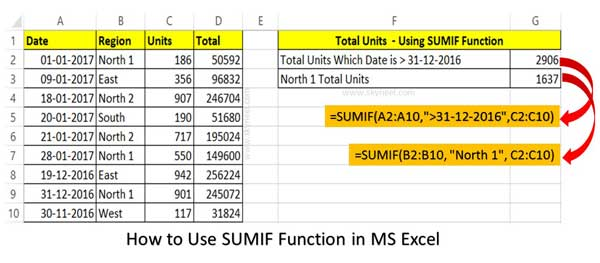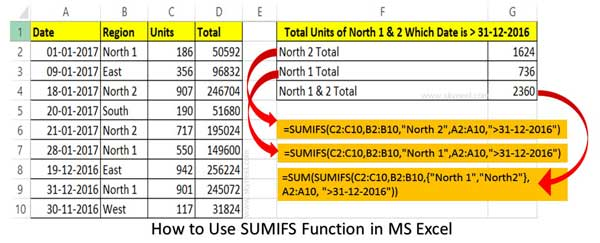# What is Difference between SUMIF and SUMIFS Function

SUMIF and SUMIFS function are used for conditionally sum of given range. SUMIF is used for single condition while SUMIFS is used for multiple criteria.

SUMIF function allows you to conditionally sum the values which match the given single criteria, While SUMIF is used to conditionally sum the values which match the multiple criteria. Most of the person little bit confused during using these functions. Today we have to discuss what is the difference between the SUMIF and SUMIFS function in Excel.

## Difference between SUMIF and SUMIFS Function

SUMIF and SUMIFS are both important functions which are located in the Math and Trig category. SUMIF function is designed to work with only one criteria and return the result which match the given condition. SUMIFS function is used to get the conditional total which match the given more than one criteria in active worksheet.

### SUMIF Function in Excel

SUM is an important function used to get the total of selected range of cells. But sometime we need to sum selected range of cells which match the given condition. In that situation you have to use SUMIF function at the place of SUM function in Excel. SUMIF is an important function of Excel which is located in the Mathematical and Trig category. This function is used to conditionally sum the specified range values which match the given condition.SUMIF(range, criteria, [sum_range])

range: It the range of continuous cells that you want to evaluated by the given criteria.

criteria: It is a condition which specifies that which items you want to add that match the given criteria in the range.

sum_range: It is an optional argument which specifies which cells to be added. If “sum_range” argument is omitted then “range” is used as a “sum_range”.

Step 1: First you have to prepare the given table in new worksheet.

Step 2: If you want to get the total units only for “North 1” region then you have to apply the given SUMIF function.

=SUMIF(B27:B35, “North 1”, C27:C35)

If you want to get the total units for all those cells which date is greater than “31-12-2016” then you have to apply the given SUMIF function.

=SUMIF(A27:A35,”>31-12-2016″,C27:C35)

### SUMIFS Function in Excel

SUMIFS is an important Excel function which is found in Mathematical and Trig category. This function allows you to add the continuous range of cells which is specified by a given set of conditions or criteria in active worksheet. If you have more than one conditions or criteria then you have to use SUMIFS function. If you have Excel 2007 or upper version then you have to use SUMIF function on active worksheet. But if you don’t Excel 2003 or lower version then you are not able to use this function in active worksheet.SUMIFS(sum_range, criteria_range1, criteria1, [criteria_range2, criteria2], …)

sum_range – It is the range of cells which you want to add which match the given conditions. All blank and text values are ignored.

criteria_range1 – It is the first range which is evaluated by the first specified criteria.

criteria1 – It is the first condition that must be matched in the criteria_range 1.

criteria_range2, criteria2, … – These are additional ranges and criteria which you can associated with them as per your need. It is an optional part. You have to specified up to 127 range or criteria as per your requirement during using the SUMIFS function.

Step 1: Create the following given table in new worksheet.

Step 2: If you want to get the total units only for “North 1” region which date is greater than “31-12-2016” then you have to apply following given formula.

=SUMIFS(C27:C35,B27:B35,”North 1″,A27:A35,”>31-12-2016″)

If you want to get the total units only for “North 2” region which date is greater than “31-12-2016” then you have to apply following given formula.

=SUMIFS(C27:C35,B27:B35,”North 2″,A27:A35,”>31-12-2016″)

If you want to get the total units of “North 1” & “North 2” region which date is greater than “31-12-2016” then you have to apply following given formula.

=SUM(SUMIFS(C27:C35,B27:B35,{“North 1″,”North 2″},A27:A35,”>31-12-2016″))

I hope after reading this difference between SUMIF and SUMIFS Function article, you can understand what is the difference between the SUMIF and SUMIFS function in Excel. If you have any suggestion regarding this guide then please write us in the comment box. Thanks to all.